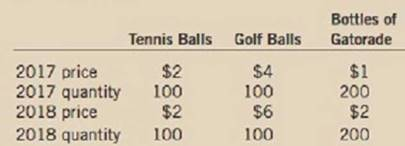Chapter 6, Problem 3PA

Chapter
Section
Textbook Problem

Suppose that people consume only three goods, as shown in this table:a. What is the percentage change in the price of each of the three goods?b. Using a method similar to the CPI, compute the percentage change in the overall price level.c. If you were to learn that a bottle of Gatorade increased in size from 2017 to 2018, should that information affect your calculation of the inflation rate? If so, how?d. If you were to learn that Gatorade introduced new flavors in 2018, should that information affect your calculation of the inflation rate? If so, how?

Subpart (a):

To determine

Measuring percentage change in price, CPI and Inflation rate.

Explanation

The percentage change in price of the good is calculated by using the following formula:

Percentage change in price =PricePresent yearPricePrevious yearPricePrevious year×100 (1)

Substitute respective values in equation (1) to calculate the percentage price change for the tennis ball.

Percentage change in priceTennis ball=222×100=0%

The percentage change in price for Tennis ball is 0.

Substitute respective values in equation (1) to calculate the percentage price change for the golf ball

Subpart (b):

To determine

Measuring percentage change in price, CPI and Inflation rate.

Subpart (c):

To determine

Measuring percentage change in price, CPI and Inflation rate.

Subpart (d):

To determine

Measuring percentage change in price, CPI and Inflation rate.

Still sussing out bartleby?

Check out a sample textbook solution.

See a sample solution

The Solution to Your Study Problems

Bartleby provides explanations to thousands of textbook problems written by our experts, many with advanced degrees!

Get Started

How do unions affect the natural rate of unemployment?

Principles of Macroeconomics (MindTap Course List)

What is a SSAE 16 report?

Accounting Information Systems

EXCHANGE RATES Use the foreign exchange section of a current issue of The Watt Street Journal to look up the si...

Fundamentals of Financial Management, Concise Edition (with Thomson ONE - Business School Edition, 1 term (6 months) Printed Access Card) (MindTap Course List)

Describe four tips for finding errors on the work sheet.

College Accounting, Chapters 1-27 (New in Accounting from Heintz and Parry)